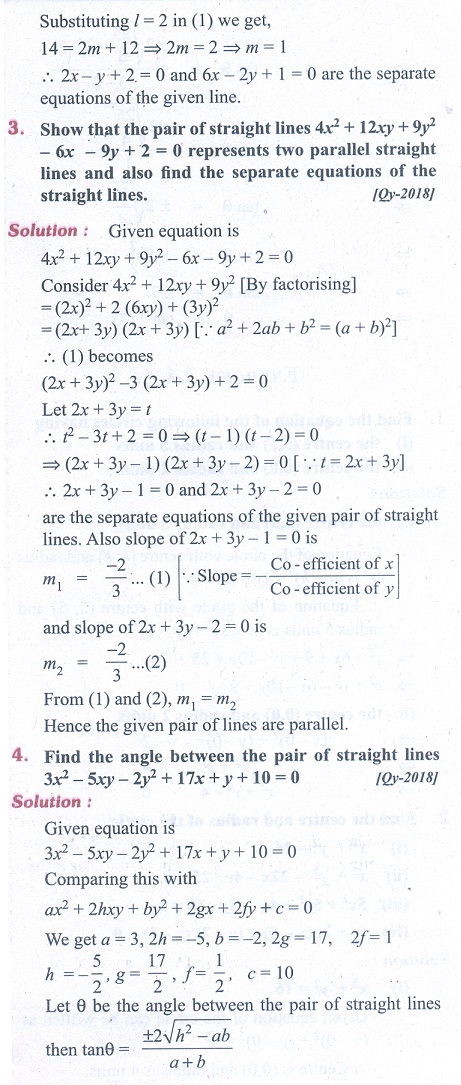Home | | Business Maths 11th std | Exercise 3.3: Pair of straight lines

# Exercise 3.3: Pair of straight lines

Business Mathematics and Statistics Book back answers and solution for Exercise questions - Analytical Geometry: Pair of straight linesTags : Problem Questions with Answer, Solution | Analytical Geometry , 11th Business Mathematics and Statistics(EMS) : Chapter 3 : Analytical Geometry
Study Material, Lecturing Notes, Assignment, Reference, Wiki description explanation, brief detail
11th Business Mathematics and Statistics(EMS) : Chapter 3 : Analytical Geometry : Exercise 3.3: Pair of straight lines | Problem Questions with Answer, Solution | Analytical Geometry